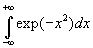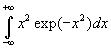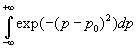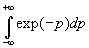# 1.[1+1+1pt] Change of variables in multiple integrals

a.                   Show that the surface element dx dy in Cartesian coordinates corresponds to r dr dj in polar coordinates by evaluating the Jacobian for this transformation of variables.

b.                  Show that the volume element dx dy in Cartesian coordinates corresponds to r2 sinq dqdrdj in spherical coordinates by evaluating the Jacobian for this transformation of variables.

c.                   Suppose a transformation of variables is given by x=u/v and y=v. Evaluate the Jacobian for this transformation.
(Hint for all three parts: Consult a calculus book on this topic.)

# 2.[1+1+2pt] Some definite integrals

a.                   Evaluate the integral.
(Hint: Evaluate the square of this integral and change from Cartesian to polar coordinates.)

b.                  Evaluate the integral.

c.                   Evaluate the integral.  (Hint : Integrate by parts.)

# 3.[1+1pt] Change of variables in a definite integral

a.                   Consider the integralwhere p0 is a real number.  Show that this integral is equal to.

b.                  Explain under which conditions two definite integrals are equal when a change of variable x’=x-x0 occurs (x0 is a real number).